407 493 6601

# Precalculus - Right Triangle Trigonometry

## Right Triangles [Recap]

• A right triangle is a special type of triangle in which the measure of one angle is exactly $90°$. The measure of the other two angles sums up to $90°$.
• Each side of a right triangle has a name, namely - hypotenuse, altitude (perpendicular), and base.
• The largest side of any right triangle is termed a hypotenuse and is always opposite to the right angle.
• The other two sides forming the right angle are called base and altitude (perpendicular).
• A right triangle is shown in the figure below.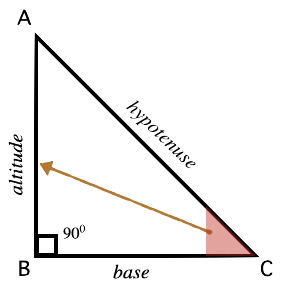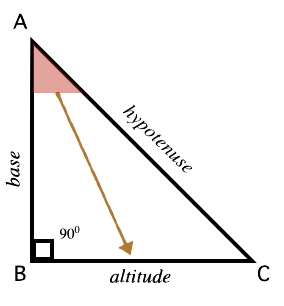• In the given right triangle $ABC$, side $AC$ opposite to the right angle is the hypotenuse.
• Notice that the altitude of a right triangle is defined relative to an angle. For example, relative to $\angle C$ side AB acts as altitude. Relative to $\angle A$, side BC acts as altitude.
• The side left after assigning hypotenuse, and altitude is designated as a base.
• A right triangle obeys Pythagoras' theorem i.e ${\left(hypotenuse\right)}^{2}={\left(base\right)}^{2}+{\left(altitude\right)}^{2}$.

## Trigonometric Ratios

• In a right-angled triangle, the ratios of sides concerning any of its acute angles are known as trigonometric ratios of that particular angle.
• In total, there are six trigonometric ratios.
• Consider right triangle ABC (as shown in the figure below) right angled at B, the trigonometric ratios of $\angle A$ are defined as follows :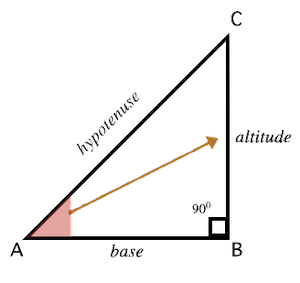sine of $=\frac{BC}{AC}$

cosine of $=\frac{AB}{AC}$

tangent of $=\frac{BC}{AB}$

cotangent of $=\frac{AB}{BC}$

secant of $=\frac{AC}{AB}$

cosecant of $=\frac{AC}{BC}$

• The trigonometric ratios defined above are abbreviated as and  respectively.

## Trigonometric ratios of complementary angles

• A pair of angles whose sum is $90°$ called complementary angles.
• In a right-angled triangle, the two acute angles are always complementary.
• Thus, for a right-angled triangle, as shown in the figure: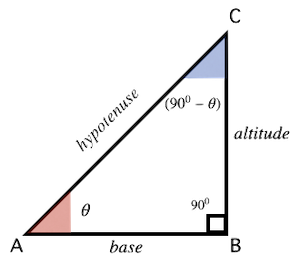## Solved Examples

Example 1: Find the values of  and  for the right triangle as shown in the figure.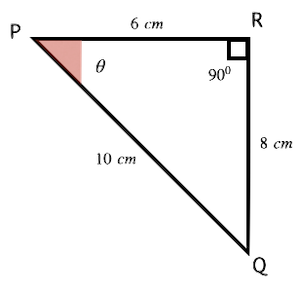Solution: For the angle '$\theta$',  and . Thus,

$=\frac{8}{10}=\frac{4}{5}$

$=\frac{6}{10}=\frac{3}{5}$

$=\frac{8}{6}=\frac{4}{3}$

Example 2: In a $△ABC$, right angled at B, if $\mathrm{sin}A=\frac{1}{\sqrt{3}}$, then find the value of .

Solution: It is given that  but . From here, we can conclude that  and , where $k$ is any positive number. Let us now draw the triangle with the known data.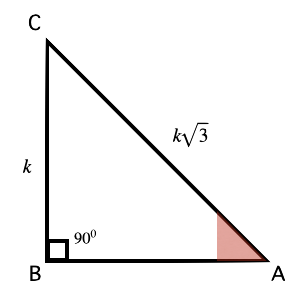Using the Pythagorean theorem, we get,

${\left(base\right)}^{2}={\left(AB\right)}^{2}={\left(k\sqrt{3}\right)}^{2}-{\left(k\right)}^{2}$

or,  ${\left(base\right)}^{2}={\left(AB\right)}^{2}=3{k}^{2}-{k}^{2}=2{k}^{2}$

so,  $base=AB=k\sqrt{2}$

Now, $=\frac{k\sqrt{2}}{k\sqrt{3}}=\frac{\sqrt{2}}{\sqrt{3}}$

similarly, $=\frac{k}{k\sqrt{2}}=\frac{1}{\sqrt{2}}$

Therefore, $\frac{1}{\sqrt{3}}×\frac{\sqrt{2}}{\sqrt{3}}×\frac{1}{\sqrt{2}}$$=\frac{1}{3}$

Example 3: In a right triangle if , find the value of the acute angle $\theta$.

Solution: It is given that . We can rewrite this expression as .

Therefore, $\theta +30°=90°-2\theta$ $⇒3\theta =90°-30°=60°$

Example 4: Find the value of .

Solution: $=1+1-1=1$

## Cheat Sheet

In any right-angled triangle, three basic trigonometric ratios defined for an acute angle '$\theta$' are:

Relationship between trigonometric ratios:

•   or    or
•   or    or
•   or    or

## Blunder Areas

• A triangle can never have two right angles.
• Trigonometric ratios apply to right-angled triangles only.